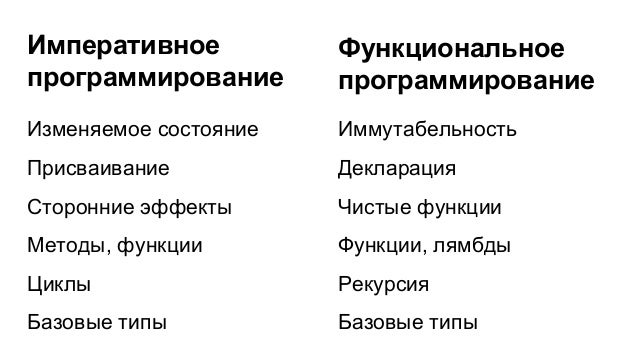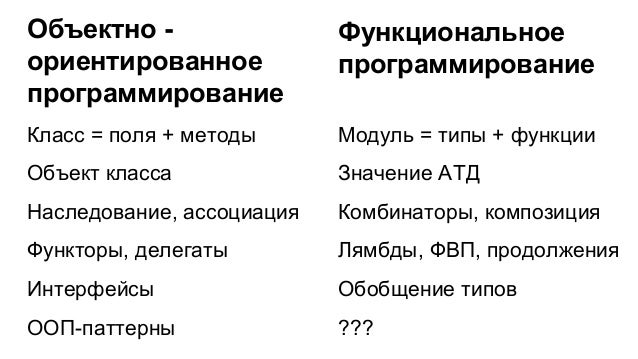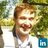Successfully reported this slideshow.Upcoming SlideShare
×

of2

Share

# Идиоматичный функциональный код

Презентация с доклада об идиоматичном ФП-коде и функциональных идиомах "зиппер", "комонада".
#1 LambdaNsk

See all

See all

### Идиоматичный функциональный код

1. 1. Идиоматичный функциональный код Александр Гранин graninas@gmail.com
2. 2. Изменяемое состояние Присваивание Сторонние эффекты Методы, функции Циклы Базовые типы Иммутабельность Декларация Чистые функции Функции, лямбды Рекурсия Базовые типы Императивное программирование Функциональное программирование
3. 3. Класс = поля + методы Объект класса Наследование, ассоциация Функторы, делегаты Интерфейсы ООП-паттерны Модуль = типы + функции Значение АТД Комбинаторы, композиция Лямбды, ФВП, продолжения Обобщение типов ??? Объектно - ориентированное программирование Функциональное программирование
4. 4. Класс = поля + методы Объект класса Наследование, ассоциация Функторы, делегаты Интерфейсы ООП-паттерны Модуль = типы + функции Значение АТД Комбинаторы, композиция Лямбды, ФВП, продолжения Обобщение типов Функциональные идиомы Объектно - ориентированное программирование Функциональное программирование
5. 5. Функциональные идиомы ● Foldable, Traversable ● Functors ● Applicative Functors ● Monoids ● Monads ● ... ● Existential types ● Lenses ● Zippers ● Comonads ● GATDs ● ...
6. 6. Функциональные идиомы ● Foldable, Traversable ● Functors ● Applicative Functors ● Monoids ● Monads ● Existential types ● Lenses ● Zippers ● Comonads ● GATDs В чем разница между понятиями “ООП-паттерн” и “ФП-идиома”?
7. 7. ООП-паттерн vs Идиома Паттерн: подход “снаружи”. Несколько классов связываются в единую систему с обобщающими интерфейсами. Идиома: подход “изнутри”. Идиома структурирует данные, обобщает и пополняет их свойства.
8. 8. Zippers http://design.vidanto.com/?p=225
9. 9. Список -- Список на АТД: data List a = Empty | Cons a (List a) myList1 = Cons 1 (Cons 2 (Cons 3 Empty)) -- Списки в Haskell: myList1 = [1, 2, 3] myList2 = 1 : 2 : 3 : [] myList3 = 1 : [2, 3] http://learnyouahaskell.com/zippers
10. 10. Zipper для списка data Zipper a = Zip [a] a [a] toLeft (Zip xs a (y:ys)) = Zip (a:xs) y ys toRight (Zip (x:xs) a ys) = Zip xs x (a:ys) extract (Zip _ a _) = a http://learnyouahaskell.com/zippers
11. 11. Zipper для списка zipper = Zip [] 0 [1..10] > toLeft zipper Zip  1 [2, 3, 4, 5, 6, 7, 8, 9, 10] > extract (toLeft (toLeft zipper)) 2
12. 12. Zip [2, 1, 0] 3 [4..10] Текущий элемент Сохраненный Контекст
13. 13. data Tree a = Empty | Node a (Tree a) (Tree a) data Direction = L | R modify :: (a -> a) -> Tree a -> [Direction] -> Tree a Дерево 1 2 5 3 1 2 50 30
14. 14. data Tree a = Empty | Node a (Tree a) (Tree a) data Direction = L | R modify :: (a -> a) -> Tree a -> [Direction] -> Tree a newTree1 = modify (*10) myTree [R, L] newTree2 = modify (*10) newTree1 [R, R] Изменение дерева 1 2 50 30
15. 15. data Tree a = Empty | Node a (Tree a) (Tree a) data NodeCtx a = LCtx a (Tree a) | RCtx a (Tree a) data TreeZipper a = TZ (Tree a) [NodeCtx a] extract (TZ (Node a _ _) _) = a Zipper для дерева
16. 16. goLeft :: TreeZipper a -> TreeZipper a goLeft (TZ (Node a l r) ctxs) = (TZ l (LCtx a r : ctxs)) goRight :: TreeZipper a -> TreeZipper a goRight (TZ (Node a l r) ctxs) = (TZ r (RCtx a l : ctxs)) goUp :: TreeZipper a -> TreeZipper a goUp = ... Zipper для дерева
17. 17. 2 35 RCtx 1 > goRight zipper TZ (Node 2 (Node 5 Empty Empty) (Node 3 Empty Empty)) [RCtx 1 Empty] 1 2 35 tree = Node 1 Empty (Node 2 (Node 5 Empty Empty) (Node 3 Empty Empty)) zipper = TZ tree []
18. 18. fromZipper :: TreeZipper a -> Tree a fromZipper (TZ cur []) = cur fromZipper z = fromZipper (goUp z) Сборка дерева RCtx 1 LCtx 2 3 5 1 2 35
19. 19. data TreeDir = U | L | R modify :: (a -> a) -> TreeZipper a -> [TreeDir] -> TreeZipper a modify f (TZ (Node a l r) ctxs) [] = TZ (Node (f a) l r) ctxs modify f z (L:dirs) = modify f (goLeft z) dirs modify f z (R:dirs) = modify f (goRight z) dirs modify f z (U:dirs) = modify f (goUp z) dirs Изменение дерева
20. 20. tree = Node 1 Empty (Node 2 (Node 5 Empty Empty) (Node 3 Empty Empty)) zipper = TZ tree [] newZipper1 = modify (*10) zipper [R, L] newZipper2 = modify (*10) newZipper1 [U, R] newTree = fromZipper newZipper Изменение дерева 1 2 5 3 1 2 50 30
21. 21. Комонады https://greyfog.files.wordpress.com/2010/02/esploso-cellular- automata.jpg
22. 22. “Жизнь” без идиом type Cell = (Int, Int) type Grid = [Cell] step :: Grid -> Grid step p = let next all [] = [] next all cur@((aX, aY) : alives) = [(x, y) | x <- lim aX, y <- lim aY, length (neighbours8 (x, y) all) == 3] ++ (next all alives) alive all cell = length (neighbours8 cell all) `elem` [2,3] in L.nub \$ filter (alive p) p ++ (next p p)
23. 23. “Жизнь” без идиом type Cell = (Int, Int) type Grid = [Cell] step :: Grid -> Grid step p = let next all [] = [] next all cur@((aX, aY) : alives) = [(x, y) | x <- lim aX, y <- lim aY, length (neighbours8 (x, y) all) == 3] ++ (next all alives) alive all cell = length (neighbours8 cell all) `elem` [2,3] in L.nub \$ filter (alive p) p ++ (next p p) 这是什么？
24. 24. “Жизнь” на монадах type Cell = (Int, Int) type Grid = [Cell] step :: Grid -> Grid step cells = do (newCell, n) <- frequencies \$ concatMap neighbours cells guard \$ (n == 3) || (n == 2 && newCell `elem` cells) return newCell http://rhnh.net/2012/01/02/conway's-game-of-life-in-haskell
25. 25. “Жизнь” на комонадах и зипперах data Universe a = Universe [a] a [a] data Cell = Dead | Alive newtype Grid = Grid (Universe (Universe Cell)) rule :: Grid Cell -> Cell rule grid | nc == 2 = extract grid | nc == 3 = Alive | otherwise = Dead where nc = length \$ filter (==Alive) (neighbours grid) next grid = grid =>> rule http://habrahabr.ru/post/225473/
26. 26. Множество Кантора на комонадах
27. 27. Правило вывода type Segment = (Float, Float) type Segments = [(Float, Float)] cantorRule :: Segment -> Segments cantorRule (x1, x2) = let len = x2 - x1 oneThird = len / 3.0 in [(x1, x1 + oneThird), (x2 - oneThird, x2)]
28. 28. Фрактал - список списков cantorGen :: Segments -> Segments cantorGen segs = concatMap cantorRule segs fractal :: [Segments] fractal = iterate cantorGen [(0.0, 0.9)] > take 2 fractal [ [(0.0,0.9)], [(0.0,0.3),(0.6,0.9)] ]
29. 29. data Layer a = Layer a comonadCantorRule :: Layer Segments -> Segments comonadCantorRule layer = cantorGen (extract layer) comonadCantorGen :: Layer Segments -> Layer Segments comonadCantorGen layer = layer =>> comonadCantorRule > take 2 \$ iterate comonadCantorGen cantorLayer [ Layer [(0.0,9.0)], Layer [(0.0,3.0),(6.0,9.0)] ] Фрактал - список слоев
30. 30. Определение комонады class Functor w => Comonad w where extract :: w a -> a duplicate :: w a -> w (w a) extend :: (w a -> b) -> w a -> w b extend f = fmap f . duplicate duplicate = extend id (=>>) :: Comonad w => w a -> (w a -> b) -> w b cx =>> rule = extend rule cx
31. 31. Простейшая комонада Layer data Layer a = Layer a instance Functor Layer where fmap f (Layer a) = Layer (f a) instance Comonad Layer where duplicate (Layer a) = Layer (Layer a) -- w a -> w (w a) extract (Layer a) = a -- w a -> a
32. 32. Layer Segments Layer Segments Layer duplicate = Layer Segments Segmentsextract = duplicate :: w a -> w (w a) extract :: w a -> a
33. 33. = Layer Segments Layer comonadRule = Layer Segments Layer Segments =>> comonadRule = =>> :: w a -> (w a -> b) -> w b comonadRule :: (w a -> b) Layer Segments Layer = fmap comonadRule =
34. 34. Спасибо за внимание! Александр Гранин graninas@gmail.com
35. 35. Зипперы - это комонады http://habrahabr.ru/post/225473/ data Universe a = Universe [a] a [a] left, right :: Universe a -> Universe a left (Universe (a:as) x bs) = Universe as a (x:bs) right (Universe as x (b:bs)) = Universe (x:as) b bs extract :: Universe a -> a extract (Universe _ x _) = x duplicate :: Universe a -> Universe (Universe a) duplicate u = Universe (tail \$ iterate left u) u (tail \$ iterate right u)
36. 36. Зиппер зипперов чисел universe = Universe [-1, -2..] 0 [1, 2..] universeOfUniverses = duplicate universe http://habrahabr.ru/post/225473/
37. 37. 1 2 35 > goLeft (goRight zipper) TZ (Node 5 Empty Empty) [ LCtx 2 (Node 3 Empty Empty) , RCtx 1 Empty] RCtx 1 LCtx 2 3 5
•#### EgorEremeev

May. 9, 2019
•#### jbmeerkat

Jul. 21, 2015

Total views

754

On Slideshare

0

From embeds

0

Number of embeds

24

9

Shares

0